Select PageWe finished the previous article with the Taxicab Number, famous in mathematics because back in 1919 one of the brightest mathematicians, H. G. Hardy, tried to console a very sick colleague, the brightest mathematician of their day, Srinivasa Ramanujan, with a number he thought was inconsequential and that he saw on a license plate of his taxicab. Ramanujan recognized it as the sum of 2 cubes in two different ways (13 + 123) and (93 plus 103).  The Creator decided to make it the number of times that the value 26 would be utilized in the composition of the Torah with 26 being the value of the Name of G-d YHVH (יהוה).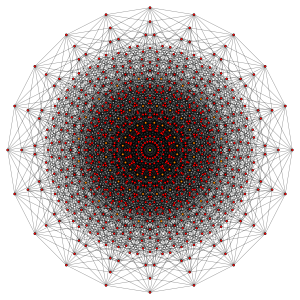That value 26 is just one of the precisely 210 different word values in the Torah. In other words, the value 26 occupies one vertex (point) of the 210 vertices in a 10-dimensional hypercube. Is this possibly coincidental given the 10-dimensional space that string theory suggests we are living in? Probably not, considering that that specific amount, or sets of word values, apply to a specific number, or sets of words in the Torah. Those specific sets, when added together, give us the simple equation 210 or 1024-word values plus the 79,976 words equal exactly (79,976 + 1024) = 81000, which in turn equals (3 x 303), 3 cubes of 303 or 27,000. It also equals (34 x 103).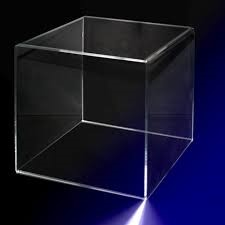The concept 26 is the value for the main Name for the Creator in the Torah, the YHVH (יהוה), utilized a total of (1729 + 91) = 1820 times. It is also integrally related to a cube, to the most basic cube, in that every 3-dimensional cube has 8 vertices or corners, 6 sides or faces, and 12 edges, and (8 + 6 + 12) = 26.

In the previous article, The Path of One; The Path of the Creator, we discussed that the Taxicab Number, 1729 was also (182091) and thus integrated into the Path of One that is based on 13, the numerical value of echad, One. What we should now point out is that the component integers in those four cubes (13 + 123) and (93 plus 103) or (1 + 3 + 1 + 2 + 3 + 9 + 3 + 1 + 3) equal 26.

Number Theory exists because numbers are concepts not fixed objects. You can temporarily assign numbers to the presence of a set of physical objects but once you remove an item in that set, the number is gone. While our universe and our entire existence is predicated on numbers, they exist only in the abstract, only in the mind of the Creator. We discussed in the previous article about Triangular Numbers that are so prevalent in the Torah how they reflect the concept that numbers never truly disappear but remain as omnipresent shadows. This is what we would call a memory, yet another concept that we cannot physically explain. These are memories in the mind of the Creator, the universal consciousness. There is nothing Man can do to change numbers or the laws of mathematics. No matter how hard we try the number 3 will always be the concept of 3, and (2 + 2) will always equal the concept of 4, no matter what symbol we use to convey that concept.

It thus behooves us to understand the best we can these abstract concepts and to accept them as gifts to help us better understand the nature of our existence. Otherwise, we are just going through life with our heads in the sand and might as well be ostriches.

That Path of One (113914551820) is based on repeatedly adding the cumulative values of repeated cycles of 13, and as we learned it is integrated with the Names of G-d in the Torah. So, it is not surprising, even though it is not mathematically causational, that (13291) = 78 = (3 x 26) or that (1331820) = 377, the 14th Fibonacci Number, whereby the two differences (78 + 377) = 455 in the Path of One, the value of the 3 Aspects, or spelled-out Names of the letters, of the Name Ehyeh (אהיה), the highest Name of the Creator used in the Torah.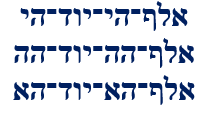While at first the reference to the concept of the 14th Fibonacci Number and thus the field of Phi (φ) is momentarily out of place, nothing is out of place within the mind of the Creator. The concept or value 455 relates to the sum of the first 6 integer cubes. That sum, (13 + 23 + 33 + 43 +53 + 63) = 441 or 212 with 21 being the value of the higher Name Ehyeh (אהיה), and 441 being the value of emet (אמת), Truth. What connects this sequence, and these disparate concepts though is that (455441) = 14 or (13 + 23 + 33 + 43 +53 + 63) + 14 = 455.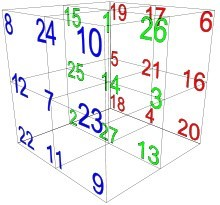And even more significantly for those who have been following this blog is that the number 14 is the central position in the Alef-bet and the Essential Cube of Creation, the Magic Cube of 42, whereby all 27 horizontal and vertical values along all 3 axes and the 10 internal diagonals all equal 42. These 27 positions coincide with the 27 ordinal values of the Alef-bet. We have covered this extensively and will be writing about it again in upcoming articles. Suffice to say for now that this means that every word in the Torah and in Hebrew can be derived from patterns and shapes within a 33 cube and that each letter in each word is bound to the concept of the Singularity of 42 in at least 3 different ways.

By the way, those 6 cubes in the concept of Truth (13 + 23 + 33 + 43 +53 + 63) correspond to both the 6 bundled dimensions of Zeir Anpin and to the 6 Faces of the Essential Cube of Creation, which along with the central 14th position equal 378, the numerical value of “He and His Name are One, Echad UShmo Echad.” And each of those 6 Faces has a total value of 126 or (21 + 22 + 23 + 24 +25 + 26), the perfect representation of 6-dimensional space, the 126 cumulative vertices in all 6 dimensions.

If we allow it, the Creator will connect all the dots for us. For example, the central 14th position and its counterpart in the Alef-bet, the 14th letter Nun (נ), connects to 13 different vectors of 42 Also, the total number of vectors of 42 that the 27 letters collectively always connect with regardless of how they are used in a word is 111, as in the Name of the letter Alef (אלף), One.

Those 111 vectors total (111 x 42) = 4662 and when we add the collective 27 ordinal values or 378, which is (9 x 42), the total works out to (4662 + 378) = 5040, the exact sum of the Earth and Moon radii, 5040 miles, which is also exactly 7 factorial or 7! = (7 x 6 x 5 x 4 x 3 x 2 x 1) = (120 x 42).

Another example is found using a similar pattern and concept as above in that the cube of 26 less the square of 26 or (263262) = 1302, with 130 being the numerical value of Mt Sinai, where we received the Torah in 1313 BCE.

It is only our own stubbornness that precludes our seeing the pictures being drawn for us.

The concept of 26 defines the basic cube and when those 26 elements of the basic cube are collapsed into a single edge of a new cube and a single square face is removed or lifted off that cube, the value of that result is 1302. To us, a cube is like a block that even babies can stack. To the cosmos, or the universal consciousness it is a geometric concept of a complexity beyond our comprehension.

In the previous articles we saw that the Creator besides being a Number Theorist also has a pension for all things geometrical, but we have only begun to scratch the surface. Those precisely chosen number of words are just one set of 3 quantitative elements of the Torah that equal exactly 58 or 390,625, which is 6252, and as we saw in the first article of this series 625 is the sum of the values of the 11 Odd interval letters of the Hebrew Alef-bet.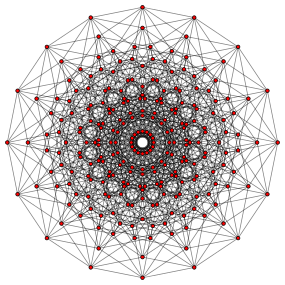Those 3 quantitative elements represent a hypercube of 8-dimensions with 5 units per edge, making a 58 octeract with a volume of 390,625.Since the octeract has 1024 edges, it aligns precisely with the 1024 vertices of the 210 Torah word value dekeract. If the simple 3-dimensional cubes have so much complexity and the entire Torah can be derived from a simple 33 cube imagine what goes on with a 10-dimensional one.

There is a special property of 390,625 or 58 and it has exactly 8 factors, each a multiple of 5 (5, 25, 125, 625, 3125, 15625, and 78125) and the sum of their 8 inverses (1/5, 1/25…) equals 1024 x 105. While the 58 elements and the 210– or 1024-word values can take on specific geometric shapes in 8 and 10 dimensions, they also form networks of symmetric matrices.

That value 1024 that keeps coming is the also the square of 32 or 322, which has been known since antiquity to be aligned with the 32 Names Elohim (אלהים) in the Torah’s first chapter, the 32 Names Elohim (אלהים) of Creation, which form the 32 vertices of the 25 or 5-dimensional Hypercube, and which the ancient Kabbalists called the 32 Paths to Wisdom.

Now, it would be amazing if those 58 or 390,625 quantitative elements also would have worked out to the sum of 2 cubes, but they do not. They are off by 30 from the sum of (523 + 633), as in the ordinal value (30) of the Name of One, Alef (אלף) and the square root of (103102), but we can assume the Creator had other intentions when He designed the Torah other than matching license plates. Nonetheless, the sum of (113 + 733) is off from precisely 390,625 by the square root of 3. To be clear, the equation is (113 + (73 + 3/102)3) = 390,625.  What makes this seem purposeful is that the square root of 3, while always significant in mathematics, is also the diagonal of a Cube, specifically the Cube of 13 whose volume is One, and moreover, the gematria value of the Torah’s first verse (Genesis 1:1) that relates simply and accurately to both Pi (π) and Phi (φ) is the 73rd Triangular Number, the sum of the integers from 1 to 73.

The second of those 58 quantitative elements are the 5845 verses. When we separate out the 13 verses of the 10 Commandments, about which we will soon explain in another upcoming article why they are a special separate entity, we are left with 5832 or 183 verses, a cube of 18 per edge, which may be related to the 1800 YHVH (יהוה) in the 3rd phase of the Torah, and given what we have seen about open cubes, to the result of (3332) = 18.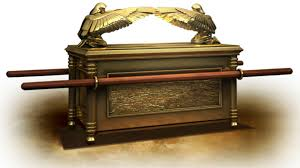Moreover, it is not a coincidence that there are 5 Books of Moses, or that those 58 quantitative elements also relate to the 28 hypercube in that 1/28 = .00390625, a fact the Creator wanted us to be well aware since the gematria of the Ark of the Covenant (הארן) is 256 or 28, and this is where Moses was told to store the Torah. The Ark (הארן) is the inverse of the Torah in that 58 = 390,625 and 1/28 = .00390625, thus 108/The Ark = The Torah.

We may not be meant to ever understand these concepts fully, but we are meant to grasp that the Torah is our entrance to the universal consciousness and that every aspect of our existence is accessible through it.

These are not the only quantitative elements in the Torah and in addition to the 58 words, verses, and letters, we have the matrix of 248 columns by 42 rows that each Torah is scaffolded on. Taking out the 16 blank rows between the Books, there are exactly 223 rows and columns, a cube of 22, as in the 22 Names of the Hebrew Letters.

The 27 Hebrew letters wrap up into a 33 cube. The 5832 verses fold up into a 183 cube, which is 216 or 63 cubes of 33. The rows and columns form a matrix that folds into a 223 cube. The letters, words and verses in the Torah form a 58 hypercube that fits in the 28 hypercube Ark. The word values in the Torah form a 210 hypercube while the word values and number of words form 3 cubes of 303.  Like triangles and Triangular Numbers, to the Creator cubes are a concept that unfolds from multiple dimensions at once. What we see is a projected snapshot of a process. Indeed the 401273 words, verses, letters, rows, and columns in the Torah reflects the symmetry of the 248 dimensions of the E8 Lattice.

Altogether the 58 words, verses, and letters plus the 223 rows and columns = 401,273 words, verses, letters, rows, and columns for the 5 quantitative Elements. We keep emphasizing quantitative because they can be physically counted and thus cannot be open to interpretation, not even after thousands of years.  Also, because their solid structure stands as proof that the Torah was given to Man as a whole complete entity, as was the Alef-bet, the key to deciphering it.  The alternative is that ancient Man not only had a profound understanding of the mathematics of the Cosmos and extraordinary computational skills, but was also capable of keeping track and organizing hundreds of thousands of letters, words, verses, word values, Names, paragraphs, chapters, and Books to form specific overlapping layers and networks of Cubes, Triangles, and hypercubes, and that is without even considering the gematria ciphers that give the Torah infinite complexity. According to archeologists they would have done this with sheafs of reeds and mounds of stones while trying to fend for themselves in the desert.

The reason this number for the 5 elements is so astonishing is that 401,273 is precisely 248,000(φ) or 248,000 times Phi (φ) packets of information for the 5 Books of Moses, whose 5 Names equal 2480, and whose 210 value positions total 524800.

Since all Torah knowledge tends toward and points to Alef (One) and since the square root of 5 is at the core of Phi (φ) and of the Torah, it is not surprising that 248/√5 = 111 (Alef), which aligns with the 111 vectors of 42 that integrate the 27 Letters of the Alef-bet folded up in a 33 cube.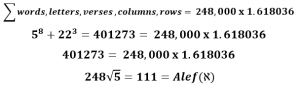We have explained in depth in our more challenging on-line Books, The Ultimate Reality Beyond the Universe and The Journey how the Torah is connected to the 248 dimensions of the E8 Lattice hyper structure and symmetry, and we will do so again in more accessible upcoming articles. Nonetheless, those 58 elements are equal to (√5)16, which is significant because Phi(φ) = (1 + √5)/2. A little basic algebra and we get √5 = (2φ1). This in turn means that the sum of the 11 Odd Letters, 625 = (2φ1)8 and that (2φ1)16 = 390,625, the sum of the 3 main quantitative elements of the Torah.  Therefore, both the 3 main elements of the Torah and the 5 main ones are directly related to Phi(φ).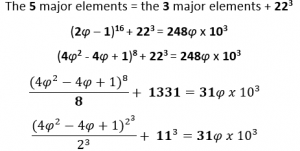As it happens 31 is the 11th Prime Number. We also saw 113 in the equation (113 + (73 + 3/102)3) = 390,625.  The ancient Kabbalists who knew how to interpret the Torah and language using the various ciphers of gematria had realized early on that there was a system of 11 sefirot or spheres, which can be translated as dimensions, and they are organized geometrically as the Tree-of-Life, specifically into 2 stacked cubes with the lower cube conforming to the 6 bundled dimensions of Zeir Anpin. The highest of those sefira/dimensions is named H’Keter, the Crown, and its gematria value is 625. The next highest is Chochma, Wisdom, and its gematria value is 73. The value 248 is not a sefira/dimension, but is the gematria value of Abraham, an energetic archetype or field and it represents 248 dimensions.

This leads us to the equation that the square root of the 3 main Torah Elements divided by the 6 bundled dimension of Zeir Anpin (625/6) is equivalent to the matrix of the 42 Rows times the 248 Columns: (625 x 102)/6 = (42 x 248). We will get more into this as we get more comfortable with the basic designs, concepts, and technology built into the Torah.

We can translate the Torah into any language we want, but only one retains the Divine and impossibly complex properties it was originally imbibed with. Nonetheless, every number and figure in the Torah is designed to convey information to us in multiple ways. That figure of 401273 elements is just another way of expressing Pi (π) in that 40/12.73 = π. And this is only the edge of the surface; every physical attribute, concept, hyper-dimensional geometry and symmetry, and mathematical constant we have and many we have yet to conceive are clearly embedded in the Torah. And they are readily available to us.

Using an ancient gematria cipher that was rediscovered as a modern Number Theory concept, we can multiple the digits in a number and divide it by the sum of the digits in that number. When we apply it to the Torah’s first verse of 28 letters and 7 words and multiple it by 28/7 we get 3.141545 or Pi (π).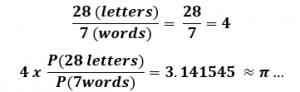When we apply to the 5 main and 3 main quantitative elements of the Torah together, we get 100042 or 6480 while the 10648 rows and columns plus the 5832 verses, in other words the cubes of 223 and 183 together equal 16480.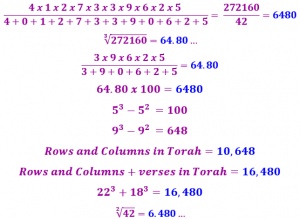Beyond and behind all the other concepts there is always the concept of 42.